Question

# a force of 227N is applied to a hydraulic jack piston that is 0.0250m in diameter....

a force of 227N is applied to a hydraulic jack piston that is 0.0250m in diameter. the piston supporting the load has a diameter of 0.175m (ignore any difference in height between the two pistons) a. what is the magnitude of the output force (N) on the hydraulic jack? b. how much mass (kg) can be lifted by the jack?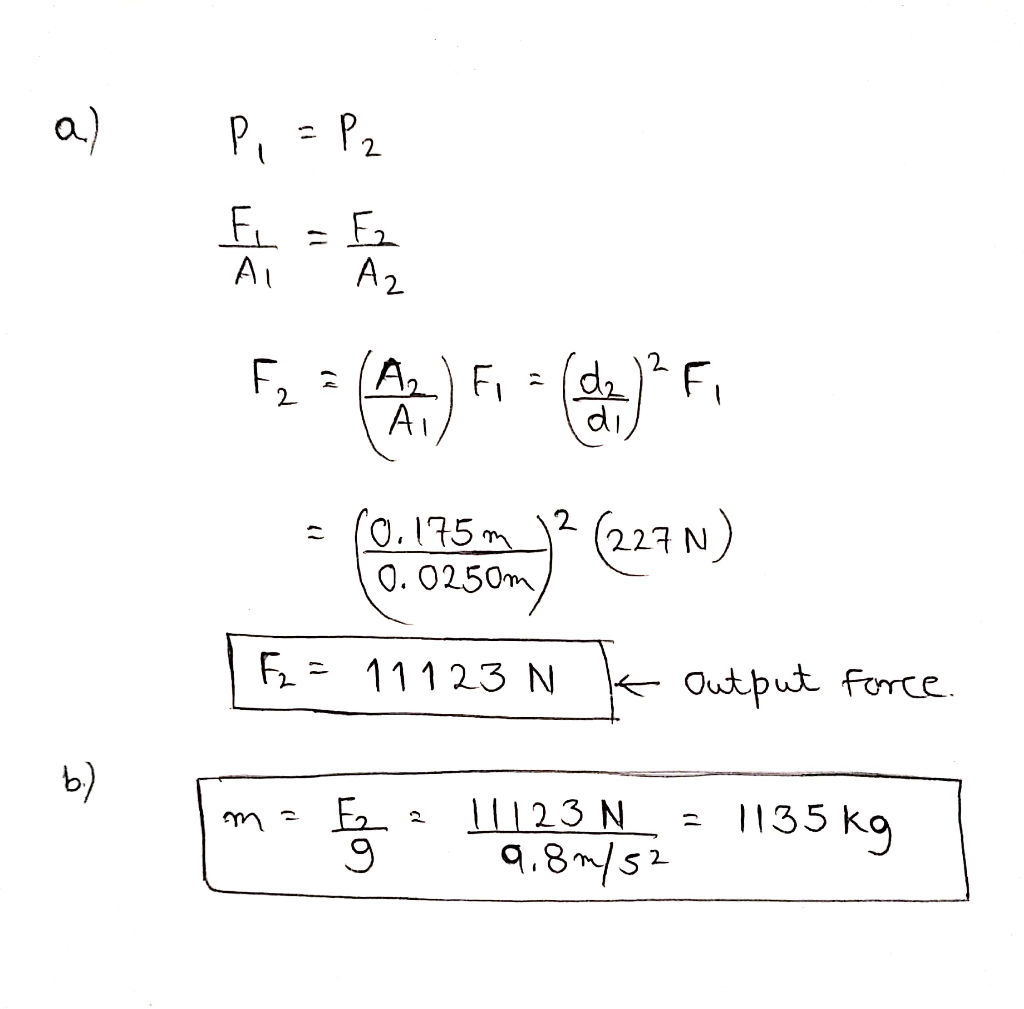#### Earn Coins

Coins can be redeemed for fabulous gifts.

Similar Homework Help Questions
• ### A hydraulic jack is made with a small piston 1.4 cm in diameter that is used...

A hydraulic jack is made with a small piston 1.4 cm in diameter that is used to move a large piston 5.3 cm in diameter. If a man can exert a force of 400 N on the small piston, how heavy a load can he lift with the jack?

• ### 2. The input and output pistons of a hydraulic jack are 4 cm and 12 cm...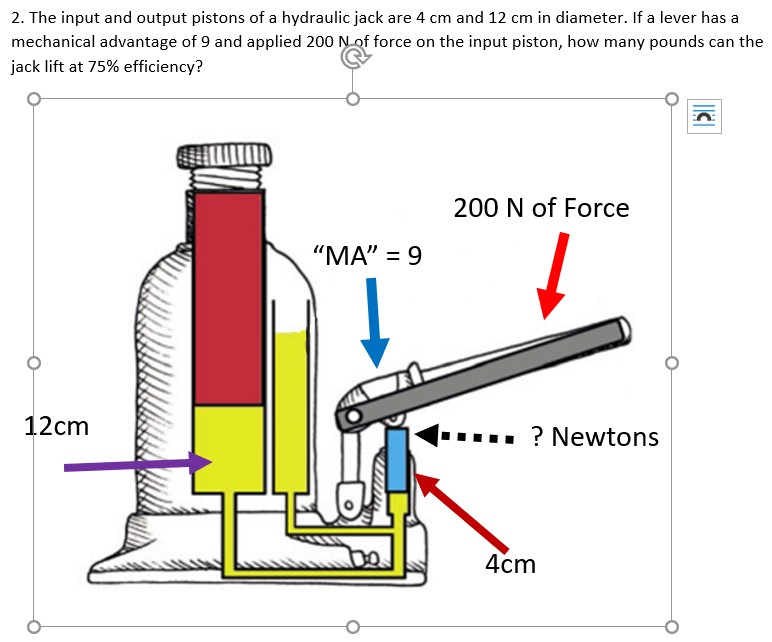2. The input and output pistons of a hydraulic jack are 4 cm and 12 cm in diameter. If a lever has a mechanical advantage of 9 and applied 200 Nof force on the input piston, how many pounds can the jack lift at 75% efficiency? 200 N of Force "MA" 9 12cm ? Newtons 4cm

• ### b) A hydraulic press (Figure 4) has a small and large piston with diameter of 50...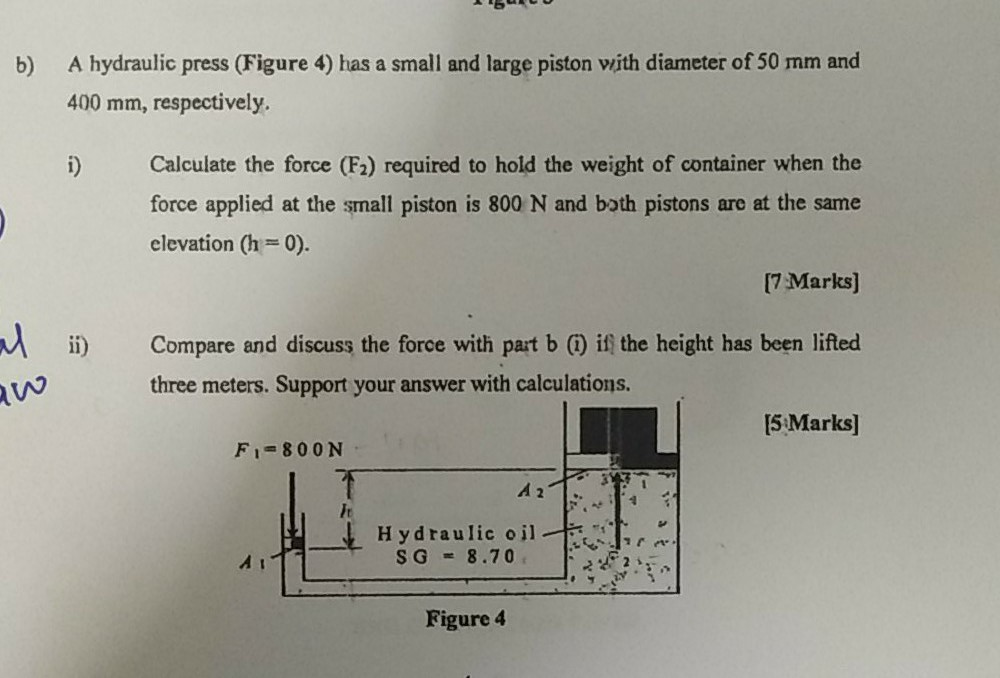b) A hydraulic press (Figure 4) has a small and large piston with diameter of 50 mm and 400 mm, respectively. i) Calculate the force (F2) required to hold the weight of container when the force applied at the small piston is 800 N and both pistons are at the same elevation (h=0). [7 Marks) i) w Compare and discuss the force with pat b () if the height has been lifted three meters. Support your answer with calculations. [5...

• ### shown in the figure below, a hydraulic system has two pistons of different diameter and uses...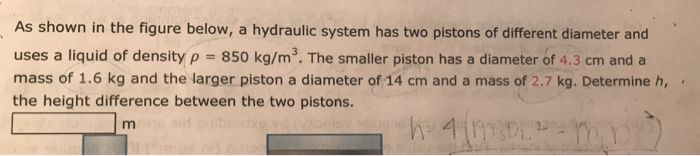shown in the figure below, a hydraulic system has two pistons of different diameter and uses a liquid of density p 850 kg/m3. The smaller piston has a diameter of 4.3 cm anda mass of 1.6 kg and the larger piston a diameter of 14 cm and a mass of 2.7 kg. Determine h the height difference between the two pistons As

• ### A Hydraulic Pump Due this Friday, Nov 13 at 11:59 pm (EST) Piston 1 in the...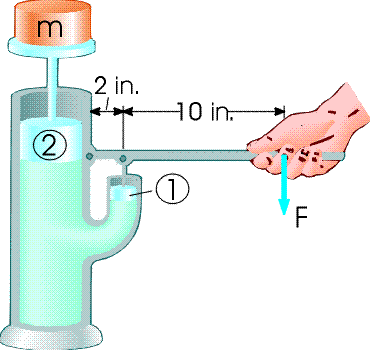A Hydraulic Pump Due this Friday, Nov 13 at 11:59 pm (EST) Piston 1 in the figure below has a diameter of 0.770 cm; piston 2 has a diameter of 3.30 cm. In the absence of friction, determine the force required on piston 1 necessary to support an object, m, with weight 840 N. (Neglect the height difference between the bottom of the two pistons, and assume that the pistons are massless). Tries 0/10 What is the magnitude of the...

• ### QUESTION 8 10 points In a hydraulic jack, a force F is applied to the small...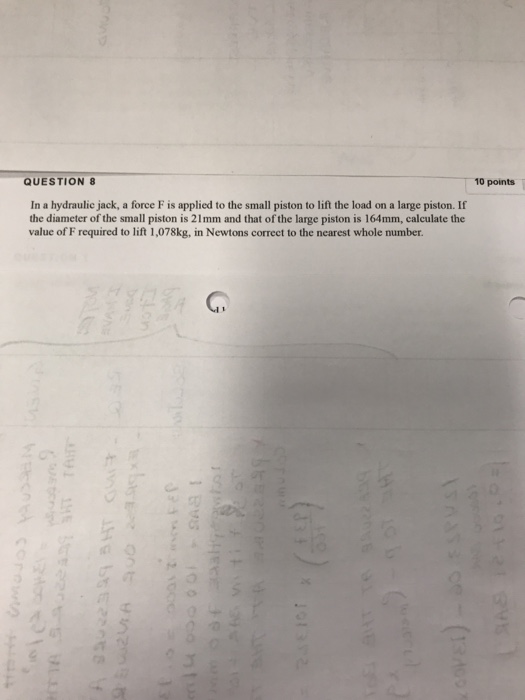QUESTION 8 10 points In a hydraulic jack, a force F is applied to the small piston to lift the load on a large piston. If the diameter of the small piston is 21mm and that of the large piston is 164mm, calculate the value of F required to lift 1,078kg, in Newtons correct to the nearest whole number.

• ### Piston 1 in the figure below has a diameter of 0.592 cm; piston kn 2 has...

Piston 1 in the figure below has a diameter of 0.592 cm; piston kn 2 has a diameter of 3.72 cm. In the absence of friction, determine the force required on piston 1 necessary to support an object, m, with weight 957 N. (Neglect the height difference between the bottom of the two pistons, and assume that the pistons are massless). What is the magnitude of the force, F, required at the end of the handle?

• ### A hydraulic press has one piston of diameter 2.0 cm and the other piston of diameter...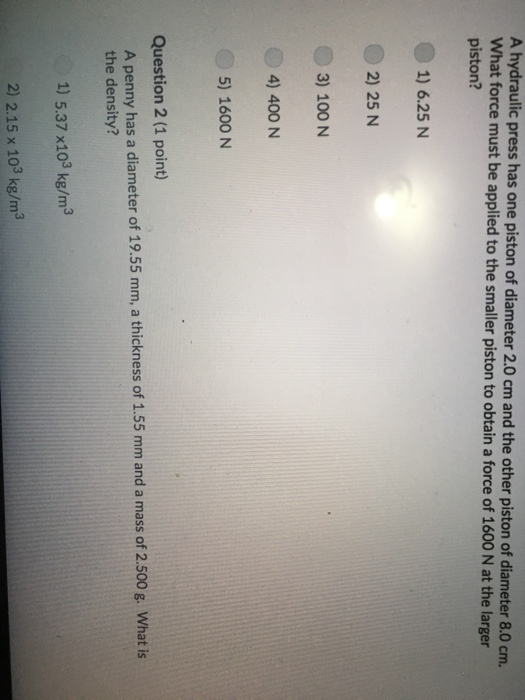A hydraulic press has one piston of diameter 2.0 cm and the other piston of diameter 8.0 cm. What force must be applied to the smaller piston to obtain a force of 1600 N at the larger piston? 1) 6.25 N 2) 25 N 3) 100 N 4) 400 N 5) 1600 N Question 2 (1 point) A penny has a diameter of 19.55 mm, a thickness of 1.55 mm and a mass of 2.500 g. What is the density?...

• ### The small piston of a hydraulic lift has a diameter of 8.0 cm, and large piston...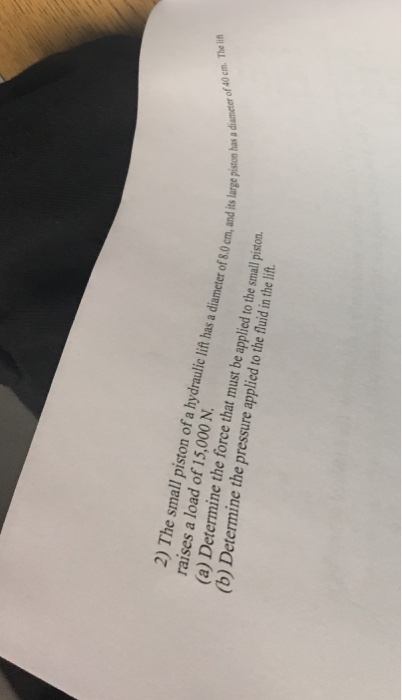The small piston of a hydraulic lift has a diameter of 8.0 cm, and large piston has a diameter of 40 cm. The lift raises a load of 15,000 N. Determine the force that must be applied to the small piston. Determine the pressure applied to the fluid in the lift.

• ### Consider the hydraulic jack represented beside. It is filled with mineral oil (a lubricating liquid). Assume...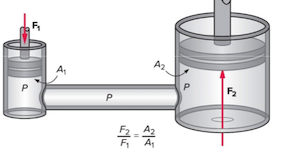Consider the hydraulic jack represented beside. It is filled with mineral oil (a lubricating liquid). Assume that a person is able to push the left piston (F1) with a maximum force of 343 N. The diameter of the piston on the left is only 2 cm and that of the piston on the right much larger at 40 cm. • A) If the piston on the right is used to lift a car, what is the highest car weight (in...